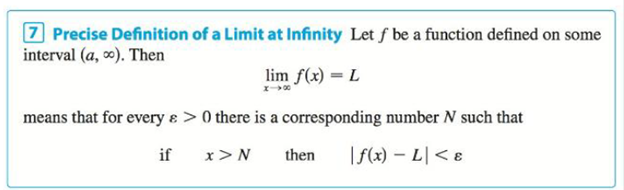Chapter 2.6, Problem 76E

Chapter
Section
Textbook Problem

(a) How large do we have to take x so that 1 x < 0.0001 ? (b) Taking r = 1 2 in Theorem 5, we have the statement lim x → ∞ 1 x = 0 Prove this directly using Definition 7.Definition 7(a)

To determine

To find: The large values of x when 1x<0.0001.

Explanation

Calculation:

Consider the inequality 1x<0.0001.

1x<0.00011x<110000

Take the reciprocal on both sides resulting by change in sign.

x>10000

Squaring on both sides

(b)

To determine

To prove: The limit function limx1x=0.

Still sussing out bartleby?

Check out a sample textbook solution.

See a sample solution

The Solution to Your Study Problems

Bartleby provides explanations to thousands of textbook problems written by our experts, many with advanced degrees!

Get Started

In Problems 1-6, find at the given point without first solving for y. 2.

Mathematical Applications for the Management, Life, and Social Sciences

What is the lowest score in the following distribution?

Essentials of Statistics for The Behavioral Sciences (MindTap Course List)

Divide: 324

Elementary Technical Mathematics

True or False:

Study Guide for Stewart's Multivariable Calculus, 8th

Explain how the mean and the standard deviation describe a distribution of scores.

Research Methods for the Behavioral Sciences (MindTap Course List)